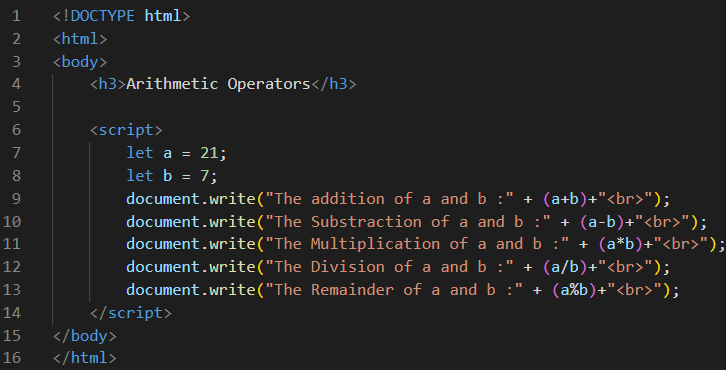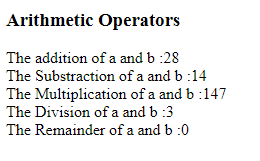JavaScript Arithmetic Operators | JavaScript Arithmetic Tutorials by WDH

# JavaScript Arithmetic Operators

JavaScript Arithmetic Operators

Arithmetic Operators are the most familiar operators because they are used every day to solve common math calculations.

The Arithmetic Operators are as shown below:

 Operator Example Operation + a + b Addition - a – b Subtraction * a * b Multiplication / a / b Division % a % b Modulo Operation(Remainder after division)

ExampleOutput